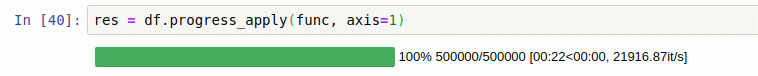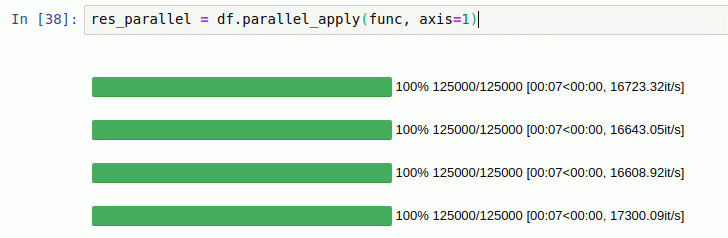# Pandarallel 一个能让你的Pandas计算火力拉满的工具

1年前 (2022-11-09) 194次浏览
 没有使用Pandarallel使用了Pandarallel• multiprocessing

• concurrent.futures.ProcessPoolExecutor()

• joblib

• ppserver

• celery

pandarallel

df.apply(func)

df.parallel_apply(func)

df.applymap(func)

df.parallel_applymap(func)

df.groupby(args).apply(func)

df.groupby(args).parallel_apply(func)

df.groupby(args1).col_name.rolling(args2).apply(func)

df.groupby(args1).col_name.rolling(args2).parallel_apply(func)

df.groupby(args1).col_name.expanding(args2).apply(func)

df.groupby(args1).col_name.expanding(args2).parallel_apply(func)

series.map(func)

series.parallel_map(func)

series.apply(func)

series.parallel_apply(func)

series.rolling(args).apply(func)

series.rolling(args).parallel_apply(func)1.准备

1. Windows 环境 打开 Cmd (开始-运行-CMD)。
2. MacOS 环境 打开 Terminal (command+空格输入Terminal)。
3. 如果你用的是 VSCode编辑器 或 Pycharm，可以直接使用界面下方的Terminal.

`pip install pandarallel`

2.使用Pandarallel

``from pandarallel import pandarallelpandarallel.initialize()``

Pandarallel一共支持8种Pandas操作，下面是一个apply方法的例子。

``import pandas as pdimport timeimport mathimport numpy as npfrom pandarallel import pandarallel# 初始化pandarallel.initialize()df_size = int(5e6)df = pd.DataFrame(dict(a=np.random.randint(1, 8, df_size),                       b=np.random.rand(df_size)))def func(x):    return math.sin(x.a**2) + math.sin(x.b**2)# 正常处理res = df.apply(func, axis=1)# 并行处理res_parallel = df.parallel_apply(func, axis=1)# 查看结果是否相同res.equals(res_parallel)``

``import pandas as pdimport timeimport mathimport numpy as npfrom pandarallel import pandarallel# 初始化pandarallel.initialize()df_size = int(3e7)df = pd.DataFrame(dict(a=np.random.randint(1, 1000, df_size),                       b=np.random.rand(df_size)))def func(df):    dum = 0    for item in df.b:        dum += math.log10(math.sqrt(math.exp(item**2)))            return dum / len(df.b)# 正常处理res = df.groupby("a").apply(func)# 并行处理res_parallel = df.groupby("a").parallel_apply(func)res.equals(res_parallel)``

``import pandas as pdimport timeimport mathimport numpy as npfrom pandarallel import pandarallel# 初始化pandarallel.initialize()df_size = int(1e6)df = pd.DataFrame(dict(a=np.random.randint(1, 300, df_size),                       b=np.random.rand(df_size)))def func(x):    return x.iloc + x.iloc ** 2 + x.iloc ** 3 + x.iloc ** 4# 正常处理res = df.groupby('a').b.rolling(4).apply(func, raw=False)# 并行处理res_parallel = df.groupby('a').b.rolling(4).parallel_apply(func, raw=False)res.equals(res_parallel)``

pandarallel

df.apply(func)

df.parallel_apply(func)

df.applymap(func)

df.parallel_applymap(func)

df.groupby(args).apply(func)

df.groupby(args).parallel_apply(func)

df.groupby(args1).col_name.rolling(args2).apply(func)

df.groupby(args1).col_name.rolling(args2).parallel_apply(func)

df.groupby(args1).col_name.expanding(args2).apply(func)

df.groupby(args1).col_name.expanding(args2).parallel_apply(func)

series.map(func)

series.parallel_map(func)

series.apply(func)

series.parallel_apply(func)

series.rolling(args).apply(func)

series.rolling(args).parallel_apply(func)

3.注意事项

1. 我有 8 个 CPU，但 parallel_apply 只能加快大约4倍的计算速度。为什么？

2. 并行化是有成本的（实例化新进程，通过共享内存发送数据，…），所以只有当并行化的计算量足够大时，并行化才是有意义的。对于很少量的数据，使用 Pandarallel 并不总是值得的。

– END –

top8488大数据 , 版权所有丨如未注明 , 均为原创丨本网站采用BY-NC-SA协议进行授权

[]• 版权声明

本站的文章和资源来自互联网或者站长
的原创，按照 CC BY -NC -SA 3.0 CN
协议发布和共享，转载或引用本站文章
应遵循相同协议。如果有侵犯版权的资
源请尽快联系站长，我们会在24h内删
除有争议的资源。
• 网站驱动

• 友情链接

• zzok